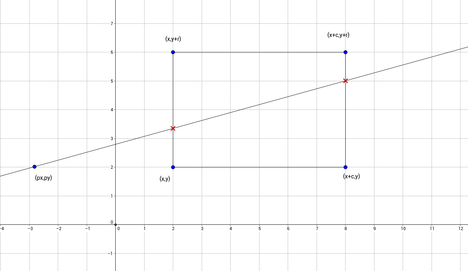# Rectangle DivisionKryptonyteThe Tough Summer Spar 201...
Limits 3.5s, 512 MB

You are given a rectangle which is parallel to the coordinate axis. The lower left coordinate is $(x,y)$ and the length of two sides are $r$ and $c$ (described in the figure). You can easily construct the rectangle by connecting these 4 points $(x,y)$, $(x+c,y)$, $(x+c,y+r)$, $(x,y+r)$ in anti-clockwise order.Figure: A line drawn form $(px,py)$ dividing the rectangle into two equal parts (according to area). Note that, the figure may not be mathematically accurate. It is used just for understanding purposes.

Now, from a given point, you have to draw a line, such that the given line divides the rectangle into two parts of equal area.

## Input

The first line of the input file contains $T$ ($1 \le T \le 500000$) which denotes the number of test cases. Each of the next lines will contain 6 floating point numbers $x$, $y$, $r$, $c$, $px$ and $py$ ($-1000000 \le x, y, r, c, px, py \le 1000000$) where $(x, y)$ is the lower left coordinate of the rectangle, $r$ and $c$ is showed in the figure and $(px, py)$ is the point from where we need to draw the line.

## Output

Output the intersected points of the drawn line and the sides of the rectangle. Print the points in lexicographical order. No two points in the output will be same.

For judging purpose error less than $10^{-6}$ will be ignored.

## Sample

InputOutput
1
0 0 6 6 3 -1
3 0
3 6

The dataset of this problem is designed in such a way that there will never be infinitely many solutions.GeometryuDebug

### Submit